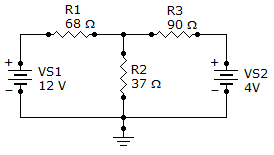# Electrical Engineering - Branch, Loop and Node Analyses - Discussion

Discussion Forum : Branch, Loop and Node Analyses - General Questions (Q.No. 3)
3.
Find branch current IR2.5.4 mA
–5.4 mA
113.0 mA
119.6 mA
Explanation:
No answer description is available. Let's discuss.
Discussion:
14 comments Page 1 of 2.

Divya N B said:   3 years ago
Multiply 68*37*90 = 226440
then 226440/68 = 3330 again 3330/2 = 1665.
226440/37 = 6120 again 6120/2 = 3060.
226440/90 = 2516 again 2516/2 = 1258.

Can anyone please explain how came 1665, 3060, and 12558?

Nisar Ahmad Stanikzai said:   8 years ago
I=112.9mA.

SURYA said:   8 years ago
(Va-12)/68)+((Va-0)/37)+((Va-4)/90) = 0.

(1665(Va-12)+3060Va+1258(Va-4))/113220 = 0.

1665Va-19980+3060Va+1258Va-5032 = 0.

5983Va = 25012.

So Va = 25012/5983 = 4. 1805 Volt but the current through the r2 resistor is 4.

180/37 = 113mA.

Pardeep singh said:   8 years ago
How is this calculation please explain?

Tamil said:   9 years ago
Correct answer is 113 mA. Thanks for giving me an idea.

Rafiqulislam said:   9 years ago

(Va-12)/68-va/37-(va-4)/90 = 0.
=> 1665(va-12)-3060va-1258(va-4) = 0.
=> 5983va = 25012.
=> va = 4.180.

Hence,

IR2 = Va/37 = 4.180/37 = 112.97 = 113mA <=

Mohammad faisal said:   9 years ago
Correct answer is 113 mA. So question is wrong.

YAHYA said:   9 years ago
Answer of this question is 113 mA.

And by the way @Selva! how can we find the branch current using the formula which you used (IR2=4.1805*37=154.678amps)?

Shubham said:   10 years ago
Kirchoff law -net current at a junction is 0.

Drop across 37 ohm resistor comes around 5v.

I equals v/r.

Which gives answer as option C.